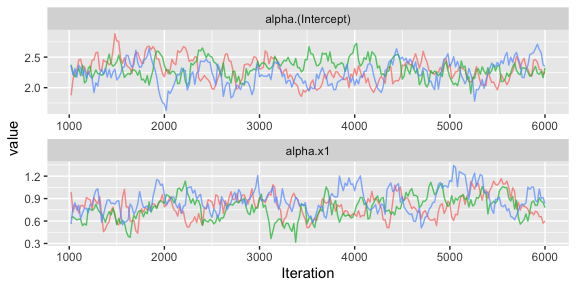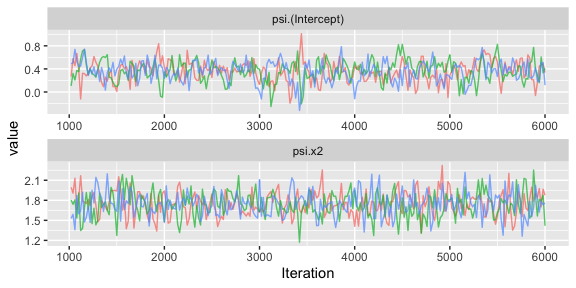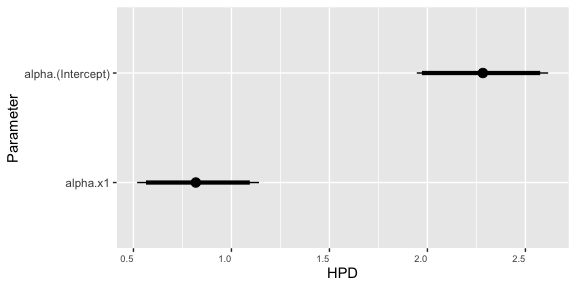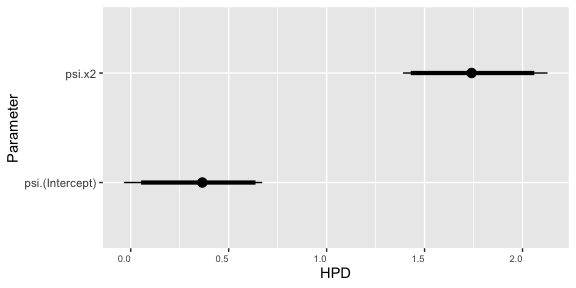# Implementation of Negative Binomial Model

#### 2021-11-22

This package illustrates the implementation of the negative binomial model for analyzing count data with overdispersion for which the level of overdisperion may vary dependent on fixed and/or random effects. As an example we consider the analysis of a simulated data set. We recommend that you first work through the vignettes for the pied flycatcher data (“pied-flycatcher-1” and “pied-flycatcher-2”) which contain further information on the structure of the package.

# Negative binomial model

The negative binomial distribution is commonly considered as a model for count data which is overdispersed relative to the Poisson distribution (i.e., the variance is bigger than the mean). The distribution is often motivated by considering independent Bernoulli trials with constant probability of success, $$p$$, and counting the number of failures that occur before a set number, $$r$$, of successes has been observed. In this case, the number of successes observed, $$Y$$, follows a negative binomial distribution with mean $E(Y)=\mu=\frac{r(1-p)}{p}$ and variance $\mbox{Var(Y)}=\frac{r(1-p)}{p^2}=\mu + \phi\mu^2$ where $$\phi=1/r$$ is the dispersion parameter.

In the implementation of the negative binomial double generalized linear mixed effects model the mean and variance for the $$i^{th}$$ observation are modelled as $g(\mu_i)=\eta_i=\mathbf x_{\mu,i}^\top \mathbf \alpha + \mathbf z_{\mu,i}^\top \mathbf \epsilon$ and $h(\phi_i)=\lambda_i= \mathbf x_{\sigma,i}^\top \mathbf \psi + \mathbf z_{\sigma,i}^\top \mathbf \xi$ with $$\mathbf \alpha$$ and $$\mathbf \epsilon$$ representing vectors of fixed and random effects for the mean and $$\mathbf \psi$$ and $$\mathbf \xi$$ fixed and random effects for the dispersion. Note that both $$\mu_i$$ and $$\phi_i$$ may take any non-negative value, and so it is natural to assume that both $$g(x)=h(x)=log(x)$$, though other link functions may be appropriate in certain situations.

# Library

First you have to load the package:

## Load package
library(dalmatian)

# Raw data

The raw data for this example is provided in the package and can be accessed with:

## Load negative binomial data
data(nbinom_data_1)

This data contains observations from a hypothetical study in which 50 individuals each observed over 30 occasions for which the observed response is a count. Let $$y_{ij}$$ denote observed count on the $$j$$th occasion for individual $$i$$. The counts for each individual are assumed to be drawn from separate, independent, negative binomial distributions with mean $\mbox{log}(\mu_{i})=\alpha_0 + \alpha_1 x_{i1} + \epsilon_{i}$ where $$x_{i1}$$ and $$z_{i1}$$ represent individual fixed and random effects on the mean and dispersion parameter defined by $\mbox{log}(\phi_{i})=\psi_0 + \psi_1 x_{i2} + \xi_{i}$ where $$x_{i2}$$ and $$\xi_{i}$$ represent individual fixed and random effects on the dispersion. For the simulation we set $$\alpha_0=\log(10)$$, $$\alpha_1=\log(2)$$, $$\psi_0=0$$, and $$\psi_1=2$$. We then generate the fixed and random effects as $x_{i1} \sim \mbox{Normal}(0,1)$ $x_{i2} \sim \mbox{Normal}(0,1)$ $\epsilon_{i} \sim \mbox{Normal}(0,1)$ $\xi_{i} \sim \mbox{Normal}(0,1).$

# Defining the Model

To illustrate the negative binomial we fit the data generating model to the simulated data. The model structure is defined through two lists specifying the fixed and random effects for the mean and dispersion components:

## Define mean and variance objects
mymean <- list(fixed = list(name = "alpha",
formula = ~x1,
priors = list(c("dnorm",0,.001))),
random = list(name = "epsilon",
formula = ~ID - 1),

mydisp <- list(fixed = list(name = "psi",
formula = ~x2,
priors = list(c("dnorm",0,.001))),
random = list(name = "xi",
formula = ~ID - 1),
link = "log")

# Running the Model with dalmatian

Once the model structure has been defined the model can be fit with the function dalmatian. The following code creates the lists of arguments supplied to jags.model and coda.samples and then calls dalmatian to run the MCMC sampler. Note that the negative binomial model is fit by specifying that family="nbinom".

## Set working directory
## By default uses a system temp directory. You probably want to change this.
workingDir <- tempdir()

## Define list of arguments for jags.model()
jm.args <- list(file=file.path(workingDir,"nbinom_test_1.R"),n.chains = 3, n.adapt = 1000)

## Define list of arguments for coda.samples()
cs.args <- list(n.iter=5000,thin=20)

## Run the model using dalmatian
nbmcmc <- dalmatian(df=nbinom_data_1,
family = "nbinom",
mean.model=mymean,
dispersion.model=mydisp,
jags.model.args=jm.args,
coda.samples.args=cs.args,
response = "y",
residuals = FALSE,
run.model = TRUE,
engine = "JAGS",
n.cores = 3,
overwrite = TRUE,
saveJAGSinput = workingDir)

# Results

Once the samples have been generated the post-processing functions can be used to examine the behaviour of the sampler and compute posterior summary statistics. These functions are described further in the help pages and in the vignettes analyzing the pied flycatcher data.

## Convergence Diagnostics

## Compute convergence diagnostics
## nbconvergence <- convergence(nbmcmc)
## Gelman-Rubin diagnostics
nbconvergence$gelman ## Potential scale reduction factors: ## ## Point est. Upper C.I. ## alpha.(Intercept) 1.047 1.15 ## alpha.x1 1.087 1.27 ## psi.(Intercept) 0.999 1.00 ## psi.x2 0.999 1.00 ## sd.epsilon.ID 1.005 1.02 ## sd.xi.ID 1.002 1.01 ## Raftery diagnostics nbconvergence$raftery
##
## Quantile (q) = 0.025
## Accuracy (r) = +/- 0.005
## Probability (s) = 0.95
##
## You need a sample size of at least 3746 with these values of q, r and s
## Effective sample size
nbconvergence$effectiveSize ## alpha.(Intercept) alpha.x1 psi.(Intercept) psi.x2 ## 100.5066 76.0233 316.0777 598.3727 ## sd.epsilon.ID sd.xi.ID ## 619.3399 640.5421 ## Traceplots ## Generate traceplots nbtraceplots <- traceplots(nbmcmc,show=FALSE) ## Fixed effects for mean nbtraceplots$meanFixed## Fixed effects for dispersion
nbtraceplots$dispersionFixed## Numerical Summaries ## Compute numerical summaries nbsummary <- summary(nbmcmc) ## Print numerical summaries nbsummary ## ## Iterations = 1020:6000 ## Thinning interval = 20 ## Number of chains = 3 ## Sample size per chain = 250 ## ## Posterior Summary Statistics for Each Model Component ## ## Mean Model: Fixed Effects ## Mean Median SD Lower 95% Lower 50% Upper 50% Upper 95% ## (Intercept) 2.28 2.28 0.18 1.95 2.17 2.41 2.62 ## x1 0.82 0.82 0.16 0.51 0.73 0.94 1.12 ## ## Mean Model: Random Effects ## Mean Median SD Lower 95% Lower 50% Upper 50% Upper 95% ## ID 1.07 1.06 0.13 0.83 0.95 1.11 1.32 ## ## Dispersion Model: Fixed Effects ## Mean Median SD Lower 95% Lower 50% Upper 50% Upper 95% ## (Intercept) 0.36 0.37 0.18 0.01 0.24 0.47 0.71 ## x2 1.74 1.74 0.19 1.40 1.64 1.88 2.13 ## ## Dispersion Model: Random Effects ## Mean Median SD Lower 95% Lower 50% Upper 50% Upper 95% ## ID 1.1 1.09 0.15 0.83 0.99 1.18 1.39 ## Graphical Summaries ## Generate caterpillar nbcaterpillar <- caterpillar(nbmcmc,show = FALSE) ## Fixed effects for mean nbcaterpillar$meanFixed## Fixed effects for dispersion
nbcaterpillar\$dispersionFixed